Ex 7.3

Chapter 7 Class 11 Permutations and Combinations
Serial order wise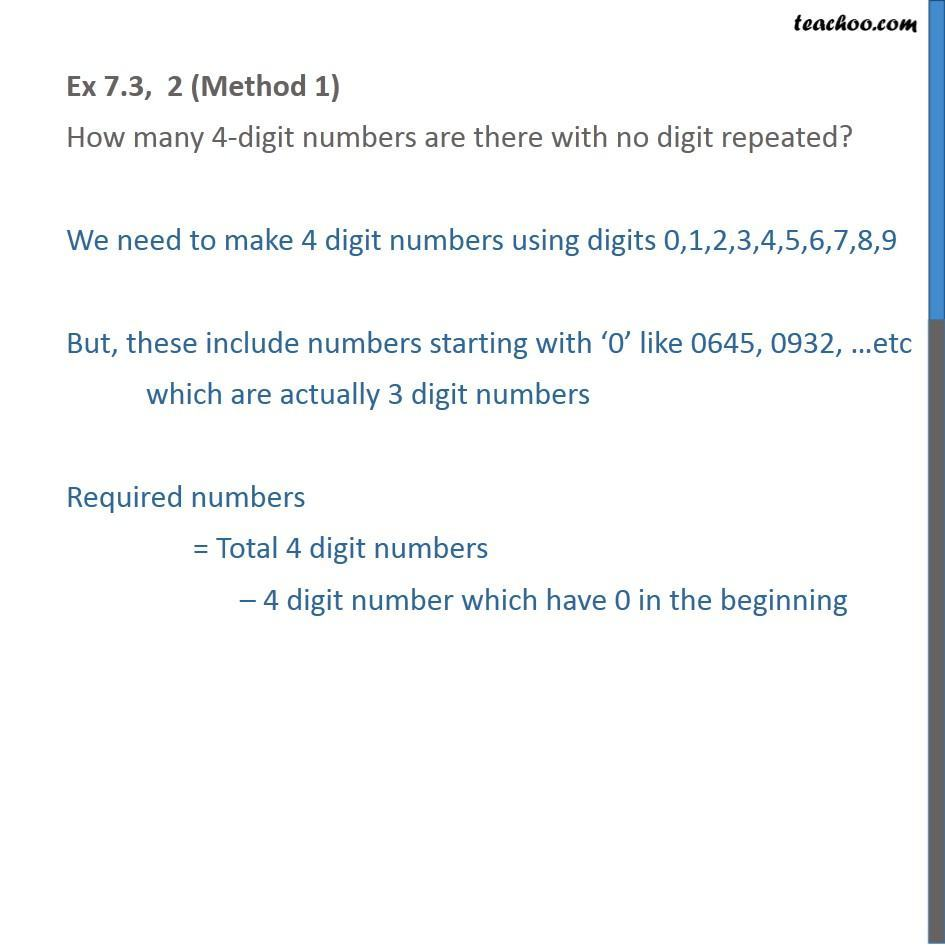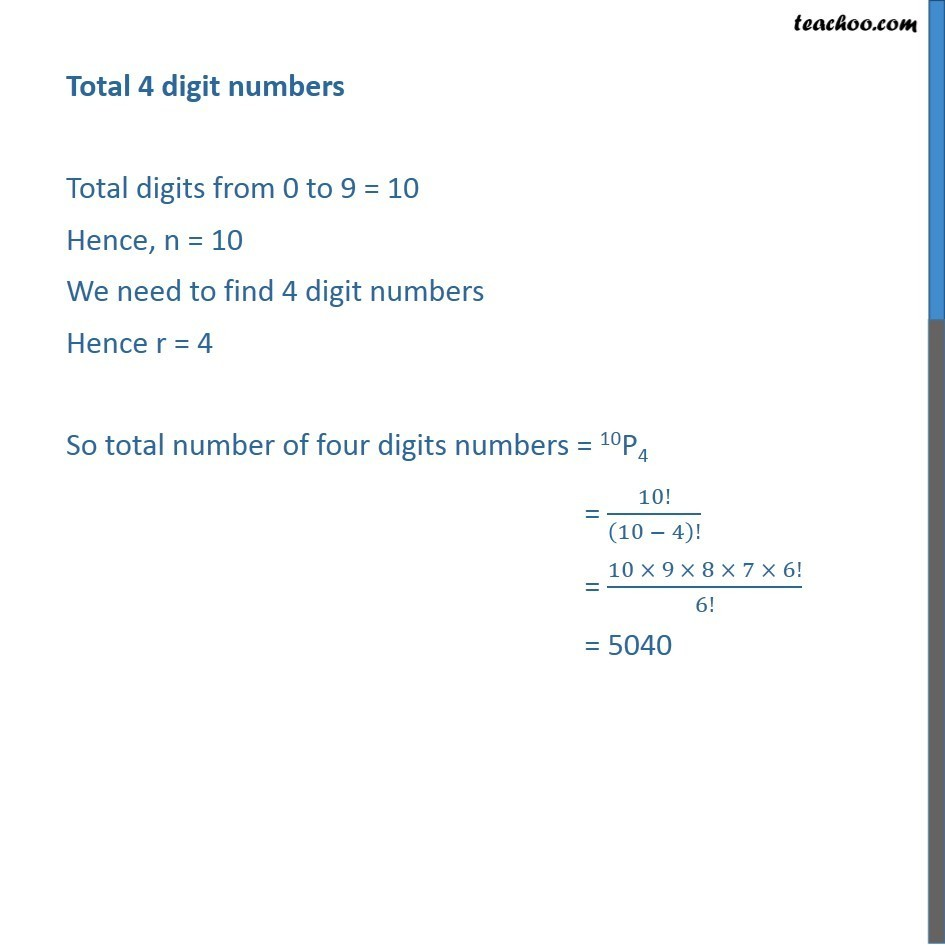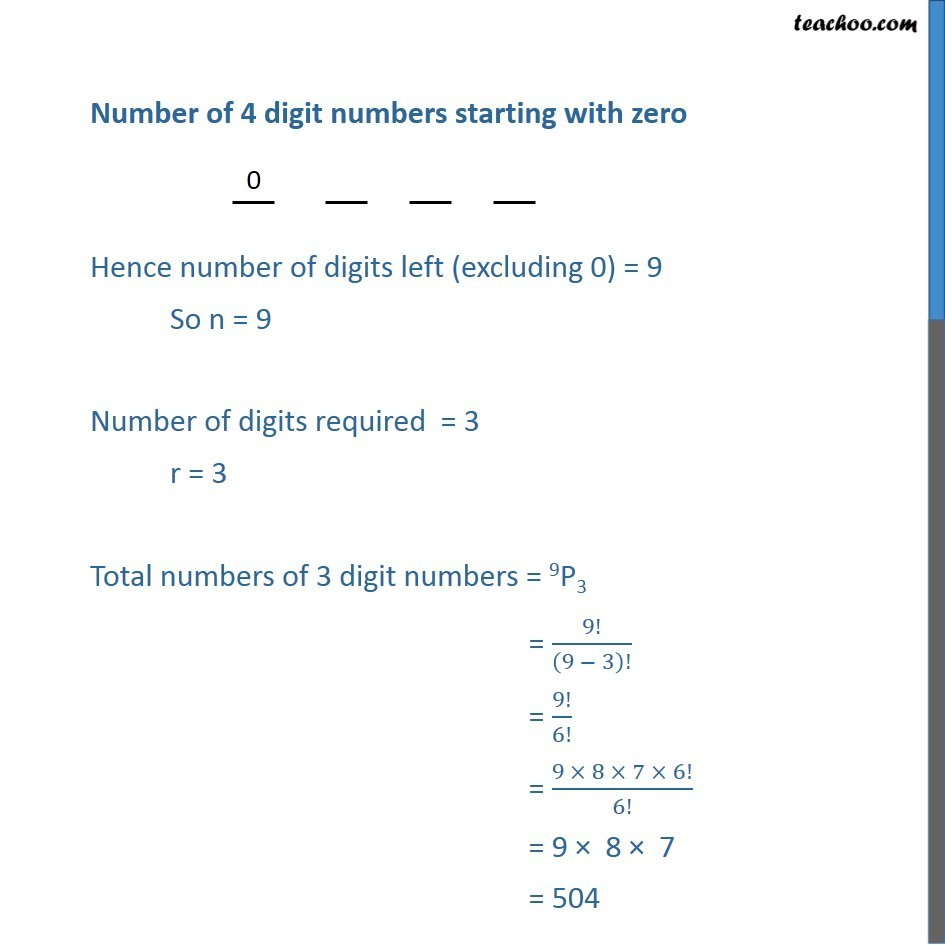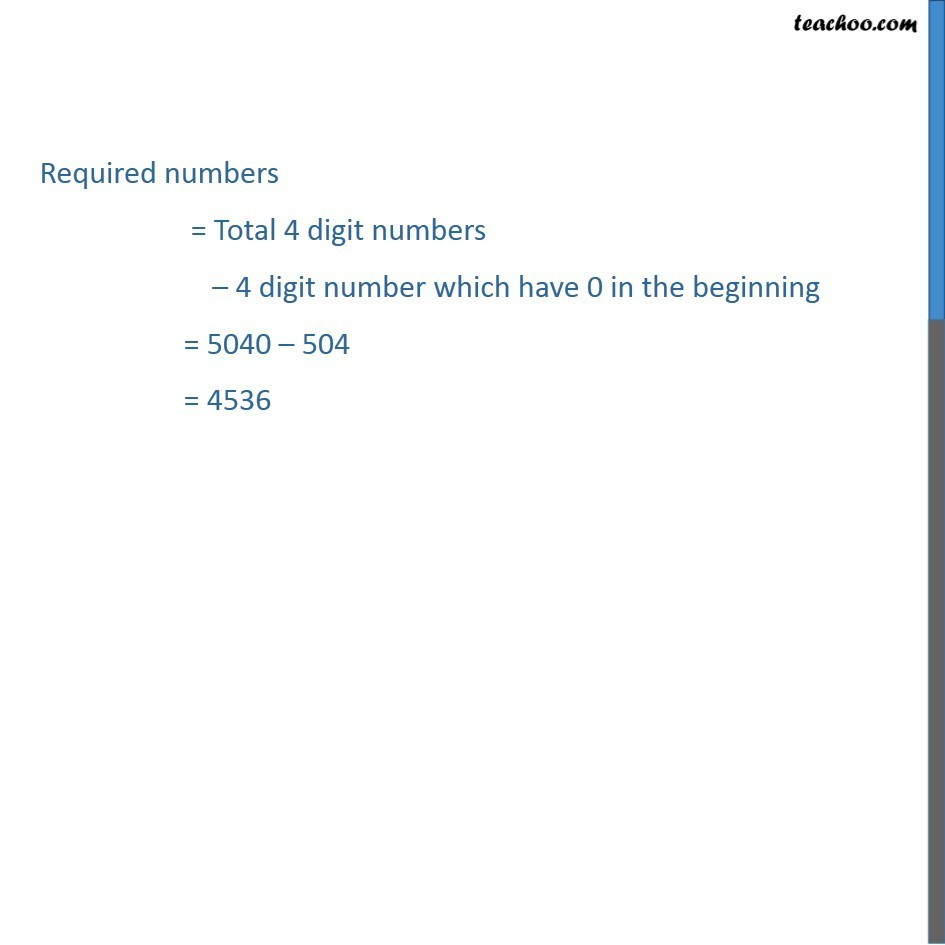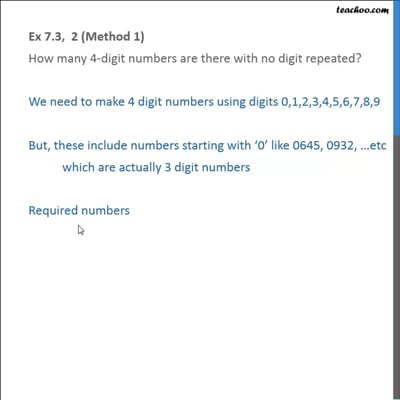This video is only available for Teachoo black users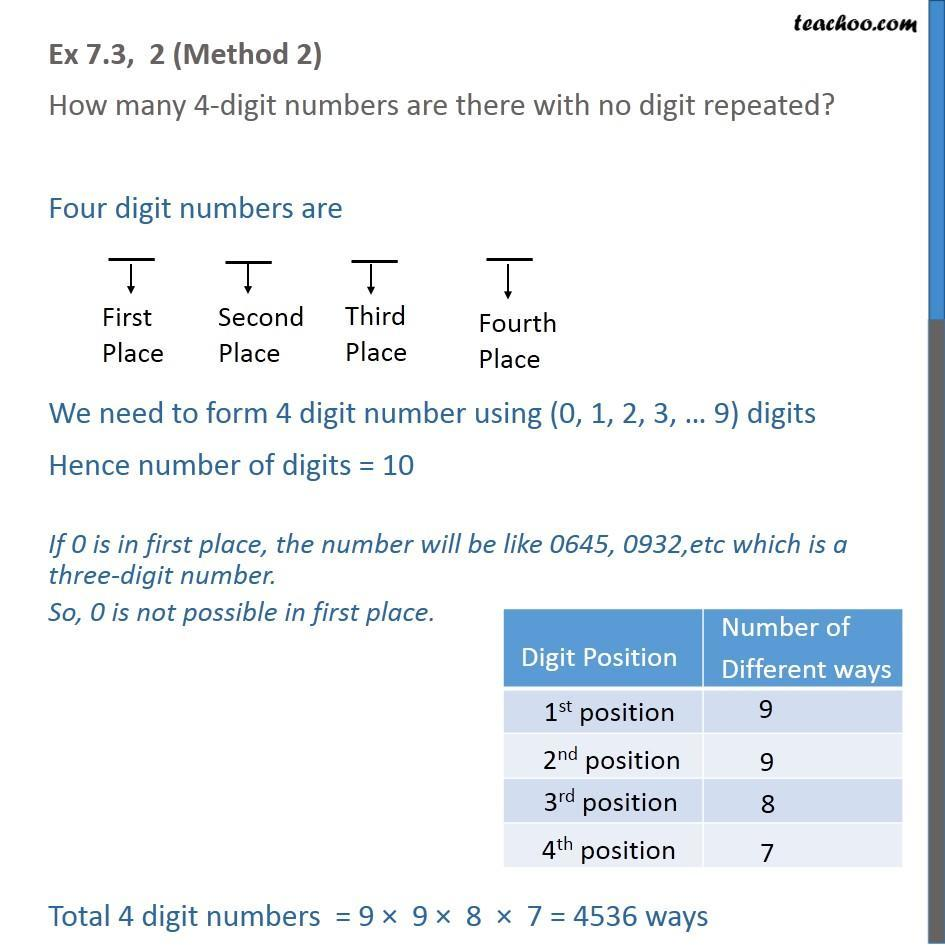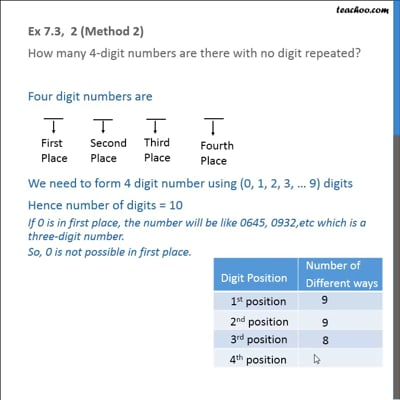This video is only available for Teachoo black users

Solve all your doubts with Teachoo Black (new monthly pack available now!)

### Transcript

Ex 7.3, 2 (Method 1) How many 4-digit numbers are there with no digit repeated? We need to make 4 digit numbers using digits 0,1,2,3,4,5,6,7,8,9 But, these include numbers starting with ‘0’ like 0645, 0932, …etc which are actually 3 digit numbers Required numbers = Total 4 digit numbers – 4 digit number which have 0 in the beginning Total 4 digit numbers Total digits from 0 to 9 = 10 Hence, n = 10 We need to find 4 digit numbers Hence r = 4 So total number of four digits numbers = 10P4 = 10!/(10 − 4)! = (10 × 9 × 8 × 7 × 6!)/6! = 5040 Number of 4 digit numbers starting with zero Hence number of digits left (excluding 0) = 9 So n = 9 Number of digits required = 3 r = 3 Total numbers of 3 digit numbers = 9P3 = 9!/(9 − 3)! = 9!/6! = (9 × 8 × 7 × 6!)/6! = 9 × 8 × 7 = 504 Ex 7.3, 2 (Method 2) How many 4-digit numbers are there with no digit repeated? Four digit numbers are We need to form 4 digit number using (0, 1, 2, 3, … 9) digits Hence number of digits = 10 If 0 is in first place, the number will be like 0645, 0932,etc which is a three-digit number. So, 0 is not possible in first place. Total 4 digit numbers = 9 × 9 × 8 × 7 = 4536 ways